Courses

# Special Theory of Relativity (Part - 2)- Classical Mechanics, CSIR-NET Physical Sciences Physics Notes | EduRev

## Physics for IIT JAM, UGC - NET, CSIR NET

Created by: Akhilesh Thakur

## Physics : Special Theory of Relativity (Part - 2)- Classical Mechanics, CSIR-NET Physical Sciences Physics Notes | EduRev

The document Special Theory of Relativity (Part - 2)- Classical Mechanics, CSIR-NET Physical Sciences Physics Notes | EduRev is a part of the Physics Course Physics for IIT JAM, UGC - NET, CSIR NET.
All you need of Physics at this link: Physics

Length contraction: Proper length

Having seen that time interval measurements in two reference frames are diﬀerent, it is natural to expect the same about length measurements too. The deﬁnition of proper length goes along the same line as that of proper time.

Deﬁnition: The Proper length of an object is its length measured in a frame in which the object is at rest. Let us compare the length of an object as measured in two reference frames. Frame S in which the object is at rest with length L, say along the x direction, and another frame S ′ in motion with respect to S along the x direction with speed v. The world lines of the object in the two frames are shown below.
Let A and B be the two events at which the two ends of the object cross the observer. In S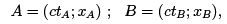(2.14)

and in S"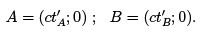(2.15)

Then the length of the object in S" is given by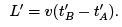(2.16)

The space time interval between A and B is the same in the two reference frames. This gives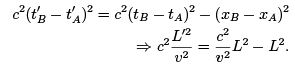(2.17)

or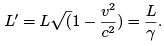(2.18)

Since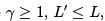i.e objects in motion appear smaller along the direction of motion.
PS: We have considered only the contraction along the direction of motion. However, it is straight forward to show (using detailed space time diagrams) that in the other two directions, i.e., the direction perpendicular to motion, there is no contraction.

Four Vectors and the ∗ product

Special relativity identiﬁes space and time not as independent entities but as two facets of a space-time continuum. Consequently, a point in space time is identiﬁed 4 coordinates, one time and three space. Thus the position vector of an object in space time is given by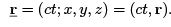(3.1)

The four-velocity vector

Every observer makes measurements in her own lab frame in her own clock - her own proper time. Evidently, thats one time on which everybody will agree. It seems natural therefore to deﬁne the four velocity of an object as the proper time derivative of its position 4-vector. However, between two observers in relative motion we know they do not measure the same time interval between two given events. Indeed, the proper time interval ∆τ is related to the time interval observed in any other frame ∆t by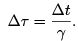(3.2)

The time derivatives in the two reference frames are related by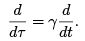(3.3)

The four velocity of a moving object is deﬁned thus: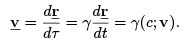(3.4)

Let’s look at each of the quantities in Eq. (3.4) carefully. The situation is the following: In a given frame (say, the rest frame) an object, whose position vector is r, is observed to be moving with velocity v. τ is the time as measured in the object’s own reference frame - its proper time. The four velocity of the object in the object’s own reference frame will be (and so will be the four velocity of anyone or anything in its own reference frame)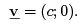(3.5)

Notice that the four velocities in Eq. (3.4) and Eq. (3.5) are one and the same, but just observed from two diﬀerent reference frames.

The ∗ product

Analogous to the "·" product of three-vectors, we deﬁne the ∗ product of two four-vectors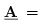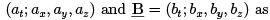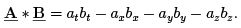(3.6)

With this deﬁnition, we notice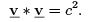(3.7)

A four vector connects two space time points. Consequently, PII can be rewritten as

• PII:The ∗ product of any two four-vectors deﬁned in space time is an invariant in any reference frame.

The four-accelaration

The four-accelaration is deﬁned as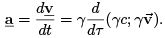(3.8)

Diﬀerentiating Eq. (3.7), we get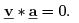Thus, a and v are always orthogonal (Note that the notion of orthogonality here is purely abstract, and should not be confused with vectors being perpendicular, as in 3-D).

3.3.1 The case of uniform accelaration

We shall look in detail an object that is uniformly accelarated, i.e., an object that feels uniformly accelarated, with accelaration say ’a’ in the x direction. From the object’s own frame it is possible to measure its accelaration (but not speed), without any reference to an external reference point. The question we adress here is, if a ground observer S were to measure the acceleration of the object, will it be the same as ’a’.
It is necessary to understand here quantitatively what we mean by a. Let the velocity of the object at some instant be v in the x direction. This speed is changing as the object accelerates.
The acceleration a is the change in velocity as seen by the object itself. Thus if we consider a reference frame S′ at a speed close to v (in the x direction), with respect to the ground observer at the same instant, then the acceleration of the object seen by S′ at that instant is roughly a. If v ′ is the velocity of the object as seen by S′, then the four-velocity of the object at that instant is (c; v′, 0, 0) (taking γ ∼ 1). The velocity being small with respect to S′, the four-accelaration is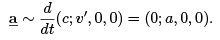(3.10)

Eq. (3.10) gives the four-acceleration as seen by S ′ around the time when the object is at speed v, and it is assumed that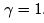. From Eq. (3.10)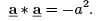(3.11)

But, the star product being an invariant, it must be same in the ground reference frame S also.
Given the special relation satisﬁed by v, Eq. (3.7), we choose the following parameterization: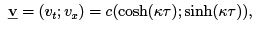(3.12)

a choice that is valid always (y and z components are zero, and hence not shown in the above).
Upon integration with respect to τ we get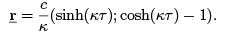(3.13)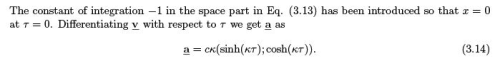Evidently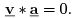The condition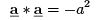(3.15)

gives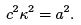(3.16)

Thus we have the ﬁnal solution,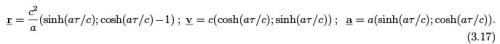The velocity as seen from the ground frame is given by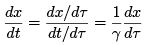(3.18)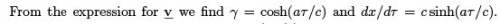as seen by the ground observer is c tanh(aτ /c). So, inspite of continued uniform acceleration, the object never reaches c in ﬁnite time.
It is important to realize here that when we say uniform acceleration, we are referring to the acceleration as seen by the accelerating object itself, in its own reference frame.

We have noted already that in going from one reference frame to another the simple velocity addition rule does not hold. In this chapter we shall ﬁnd the correct relativistic expression for adding velocities, the relations connecting space-time coordinates in two reference frames in relative motion - the Lorentz transformation relations.

Let two observers 1 and 2 in relative motion observe an object 3 in motion. Say 1 is the ground reference frame, 2 moving with respect to 1 along their common x direction with velocity v21, and let 3 also move in the x direction with speed v32 with respect to 2. Evidently the velocity of 1 with respect to 2 is v12 = −v21. The task is to ﬁnd v31, the velocity of 3 with respect to 1.
Let us write the corresponding four velocities: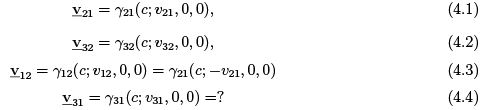where, as usual,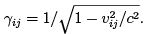To determine v31 we shall use the fact that the ∗ product of two four-vectors is invariant in any reference frame, and that the four-velocity of any object in its own reference frame is (c; 0), since in its own reference frame it is at rest. Thus, the speed of 1 in its own reference frame is zero, and consequently the four-vector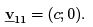(4.5)

From the invariance of the ∗ product it follows that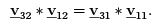(4.6)

Substituting for these four vectors from Eqs. (4.1)-(4.5), we have,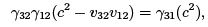(4.7)

or,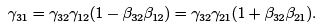(4.8)

After some simple algebra we ﬁnd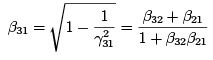(4.9)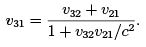(4.10)

Notice that the expression for v31 reduces to the usual Galelian expression v31 = v32 + v21 when the velocities are small compared to c.
Summary: If an object is moving with velocity v32 with respect to frame 2, and the frame 2 itself is moving with respect another frame 1 with velocity v21, then the velocity of the object with respect to 1, v31, is not the simple Galelian velocity addition law, but the expression given by Eq. (4.10), which reduces to the Galelian form for small velocities.
Not that in the above demo it is assumed that all velocivites is are in the same common x direction. If the velocities are diﬀerent the expressions are a little more complicated.

Lorentz transformations

Two observers in two diﬀerent reference frames look at the the same events diﬀerently. Their coordinates may be diﬀerent, and so could be their directions in space-time. Thus the same four vector A in space-time may be designated diﬀerently by the two observers in frames S and S" as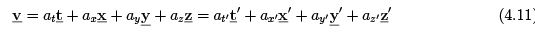We intend to ﬁnd the relations between the two sets of unit four-vectors, and the relations between the two sets of coordinates.
To do this we start with a known four vector. Recall that the four-veolcity of any object in its own reference frame is given by (c; 0, 0, 0). Thus, let us look at the four velocity of the frame S" in the two frames S and S". Let v be the velocity (say, along the x direction for simplicity) of S" with respect to S .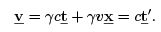(4.12)

The two vectors are the same, as they refer to the same four-vector in space time. Eq. (4.12) gives a relation between the time unit vector t′ in frame S ′ and that of the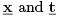in S . Now we have to determine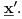. Let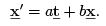(4.13)

To determine a and b we make use of the ortho normality conditions.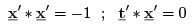(4.14)

yeild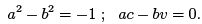(4.15)

Substituting a = bβ in the ﬁrst expression in Eq. (4.15), we have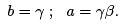(4.16)

Thus for the simple choice that the velocity is along the common x direction of the two frames, we ﬁnd the relations between the unit vectors as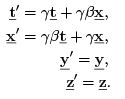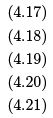The last two vectors are the same since the relative motion is along the x direction. It is now straight forward to relate the coordinates in the two frames. In Eq. (4.11) we take ∗ product with ′ on both sides. This gives,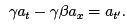(4.22)

Repeating the procedure for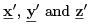(and adjusting the signs), we get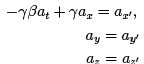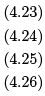In particular, the relation between the coordinates are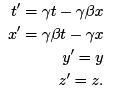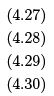As earlier, the coordinates y and z are unchanged in the two frames as the motion is only along the x direction. In general the expression connecting the two sets are They constitute the Lorentz transformation relations connecting two frames, whose axes are aligned, and their origins coincide. Such a transformation is called a pure Lorentz transformation.
The transformation matrix L in such a case is symmetric.
In general frame S" can be rotated through some constant angle with respect to S , and their origins need not coincide.

Relativistic Mechanics

The purposefulness of energy and momentum come from their conservation criterions. For an isolated system the total momentum is conserved, and so is the energy. But, will the momentum conservation hold if we were to look at the same system from any reference frames. Given that velocity addition is not the simple Galelian relation, but the expression in Eq. (4.10) (in fact more complicated for a general case), momentum conservation is tricky business as viewed from diﬀerent reference frames. In this chapter we analyze this question, and that of energy, and look at the modiﬁcations Special relativity makes to mechanics.

Momentum, as we know it, of an isolated system of particles is not conserved if we look at it from diﬀerent reference frames

Consider an isolated system of 2 particles that collide head on and perfectly elastical ly. The motion is essentially one dimensional. Let v1 and v2 be their velocities before collision and v1′ and v2′ after the collision. If m1 and m2 are their respective velocities, then the momentum conservation demands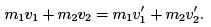(5.1)

Or, dividing through out by c,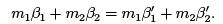(5.2)

Let us consider another reference frame S′ moving at velocity v0, with respect to the lab frame in the same direction of motion of the two particles. Let the velocities in S′ frame be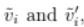i=1,2 or again dividing by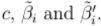. If momentum conservation holds then it must hold in all reference frames, and hence in S ′ we demand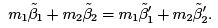(5.3)

But from the velocity addition rule in Eq. (4.9), we should have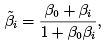(5.4)

and similarly for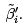Substituting in Eq. (5.3), we have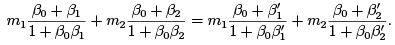(5.5)

Given Eq. (5.1), and that β0 is arbitrary in the range [0, 1], there is no way Eq. (5.5) will be satisﬁed for all β0. The momentum, as we know it, of an isolated system is not a constant!! It is easily to check along similar lines that the kinetic energy of the two particle system is not conserved either!

The four-momentum

Momentum and energy of an isolated system not being a constant as seen from diﬀerent reference frames poses a serious problem for mechanics. It means that what is an isolated system for one observer might appear to be acted upon by forces from some other inertial reference frame -questioning Newton’s laws. The resolution is that the expression for momentum and kinetic energy that we have been using is only an approximate one. We have to ﬁnd a correct expression for momentum and energy, that are conserved in diﬀerent reference frames, and reduce to the Newtonian expressions for low velocities. The answer comes in the form of the four-momentum.
Having already familiarized with four-velocity (see Eq. (3.4)), we deﬁne the four-momentum of an object as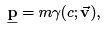(5.6)

where m is the mass of the object measured when it is at rest, or its rest mass. It is straight forward to check that the four-momentum for the system of two colliding particles is conserved in S and S".
If four-momentum is conserved in S , then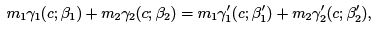(5.7)

where we have not bothered to write the y and z components, as all motion is along the spacial x direction. Being a vectorial relation, Eq. (5.7) breaks into two equations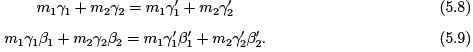We demand, in reference frame S ′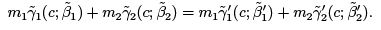(5.10)

Given Eq. (5.4), the relation between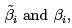it is easily seen that (Eq. (4.8) for instance)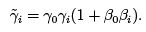(5.11)

Substituting from Eqs. (5.4) and (5.11) in Eq. (5.10), we have the temporal and spacial components as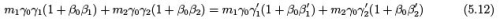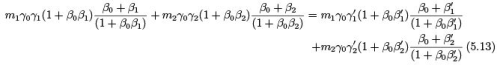Evidently, Eqs. (5.12) and (5.13) follow from Eqs. (5.8) and (5.9). Hence, we have shown that if the four-momentum is conserved in S , so will it be in S ′. And we do know that the four momentum is conserved in a reference frame where the velocities are small: in which case Eq. (5.8) is the sum of masses after and before collision, and Eq. (5.9) is the sum of Newtonian momentum.

Summary: It is not the Newtonian momentum and kinetic energy that is conserved in collision of an isolated system of two particles, but the four-momentum.

Four-momentum: Interpretation

Momentum, mass and force

The spacial part of the four-momentum,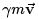reduces to the usual Newtonian momentum for small velocities. We identify this as the relativistic momentum of an object, or the correct expression for momentum. Thus the momentum of a object of rest mass m and moving with velocity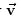is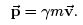(5.14)

Mass is deﬁned as momentum upon velocity, and hence we identify that the mass of an object is γ m, where m is its rest mass. With this correction, the statement of Newton’s law remains the same, except that the momentum is as deﬁned in Eq. (5.14)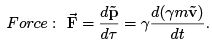(5.15)
With this deﬁnition for momentum, Eq. (5.14), it is clear that an object can never reach the velocity c. Upon acceleration its momentum will change, consistent with Newton’s law, but it starts reﬂecting in the mass than the velocity.

Energy

The most important feature of the time part of four-momentum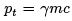in Eq. (5.6) is that it is conserved for an isolated system as seen from any reference frame. To get more clarity on this quantity, let us look at the same in familiar territory, i.e., at low velocities.
At v = 0, pt = mc, which doesn’t tell us much. For small velocities, γ can be expanded as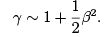(5.16)

Thus we ﬁnd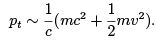(5.17)

Also,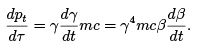(5.18)

But from Eq. (5.15), for the simple case of v along x say, we have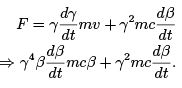(5.19)

This gives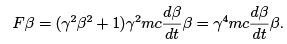(5.20)

Comparing Eq. (5.20) and Eq. (5.18), we have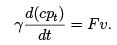(5.21)
But Eq. (5.21) is the work energy theorem in the diﬀerential form. Consequently we deﬁne the Relativistic energy as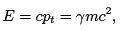(5.22)

following the three facts we have collected: a) It is conserved for an isolated system, in any reference frame, b) It goes like the kinetic energy plus a rest energy mc2 for small velocities, and c) it obeys the work energy theorem.
Thus the four momentum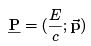(5.23)

incorporates in it both the conservation of momentum and energy. Note that the conservation of either of Eq. (5.12) and Eq. (5.13) require both Eq. (5.8) and Eq. (5.9). Thus the four dimensional nature is indispensable. Form Eq. (5.6) it follows that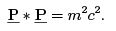(5.24)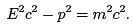(5.25)

The quantity E − mc2 will be deﬁned as kinetic energy K . Thus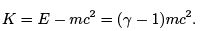(5.26)

Offer running on EduRev: Apply code STAYHOME200 to get INR 200 off on our premium plan EduRev Infinity!

159 docs

,

,

,

,

,

,

,

,

,

,

,

,

,

,

,

,

,

,

,

,

,

,

,

,

;